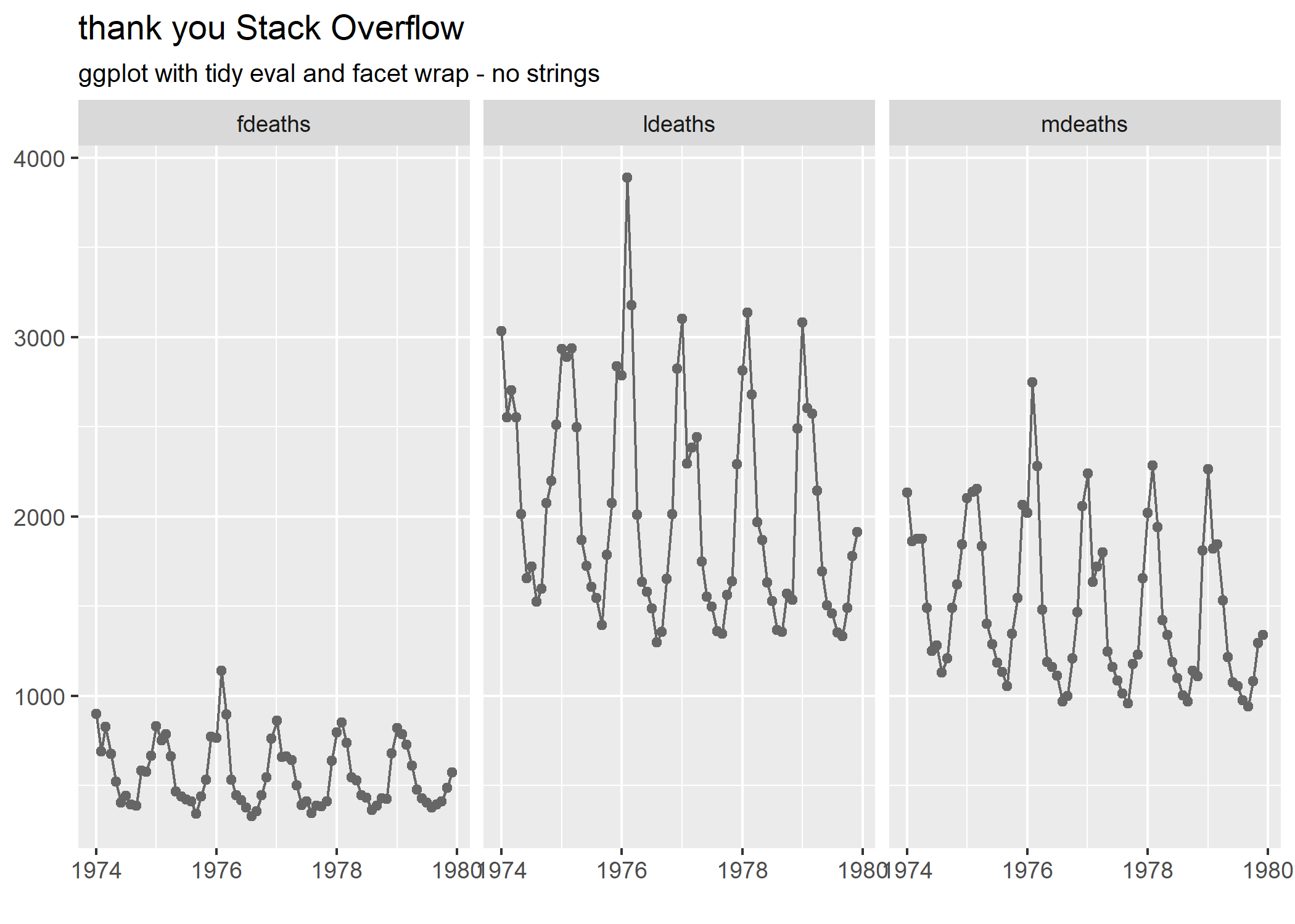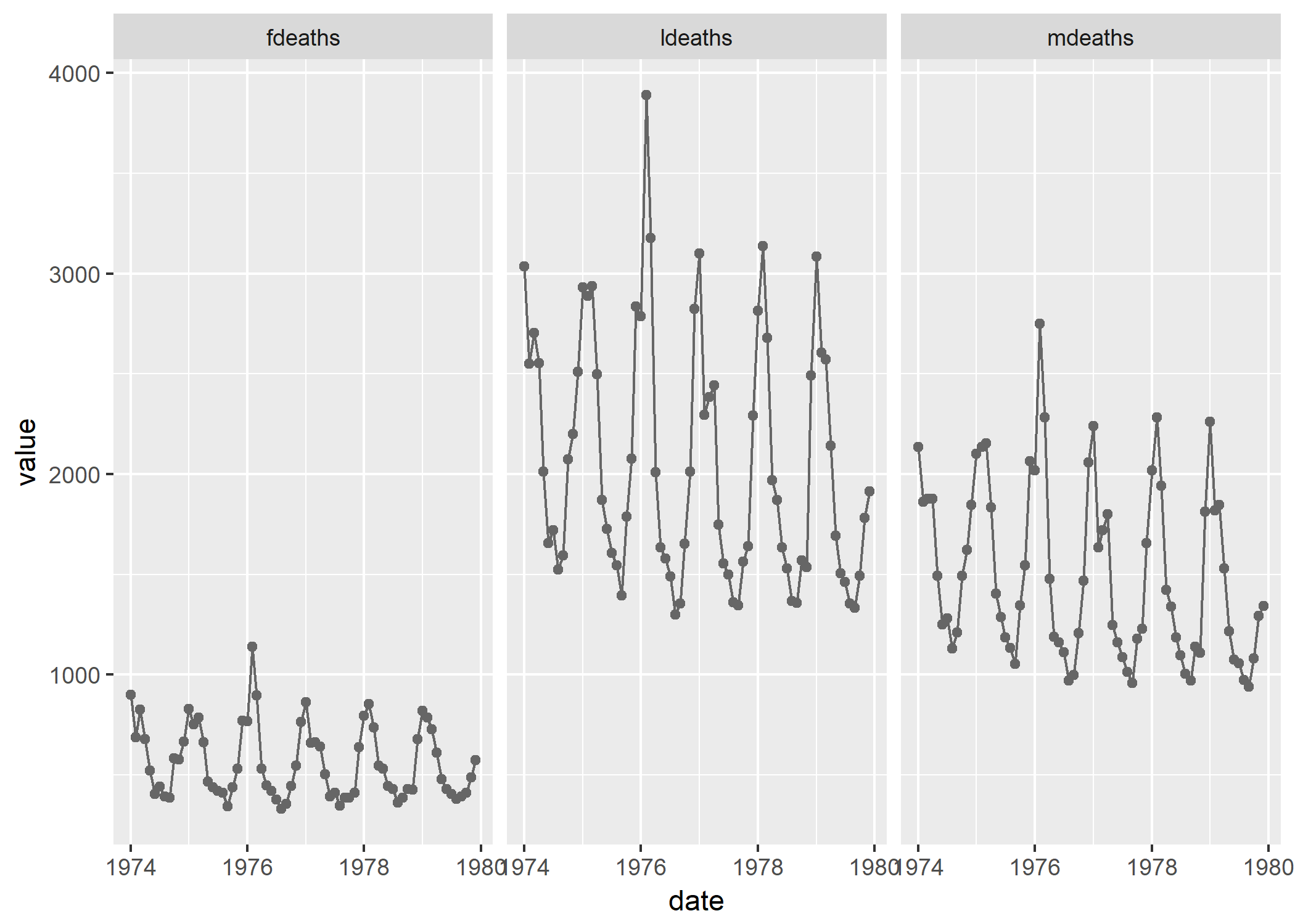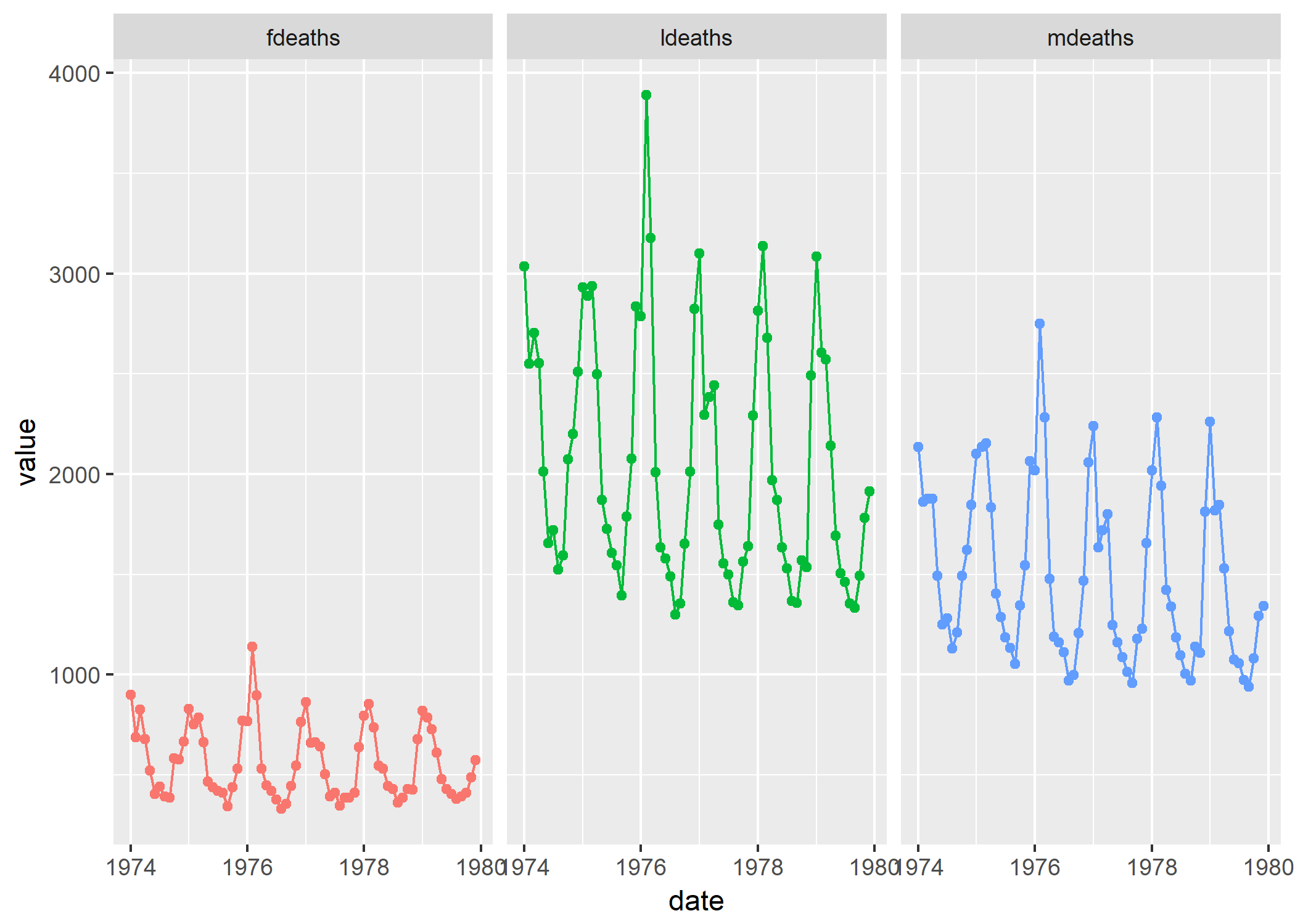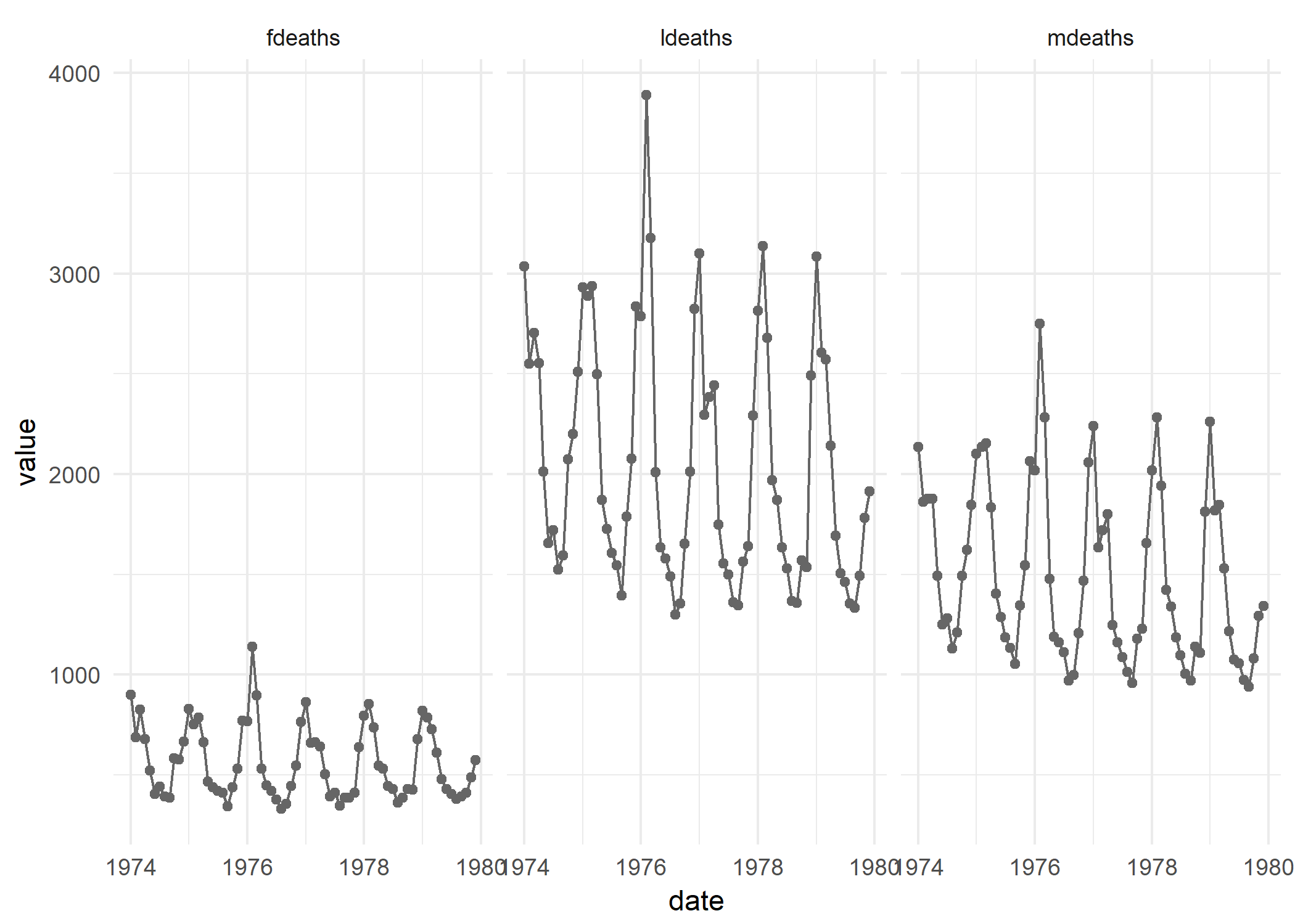# No strings attachedcreating a faceting function with bare variable names in ggplot2

I’m working on a package of dplyr and ggplot2 functions that I can use on a day to day basis to plot data over time. I’ve done some basic experimenting with my preferred ggplot2 theme settings (highlandrthemes - it’s a thing), and the next stage is to get some commonly used plot structures sorted out so that I don’t have to go hunting around for code all the time.

As I’ve been getting to grips with tidy evaluation, I wondered if I could create a ggplot2 function that would allow me to input “bare” variable names instead of inputting them as a string in the function call. I’m not a huge fan of piping direct from dplyr straight into ggplot2 but I figured if I could suss out how to create these functions, I would have the ability to pipe or not as the case required. If I get my package working properly, there will be a master function that will use tidy eval for dplyr based data munging which will feed into ggplot2.

The main requirement is having a grouping / faceting variable defined so that I can have my plots facet_wrap(ed) the way I want. As it turns out, the “~” in the facet_wrap() call is a bit pesky, to say the least.

Here’s the (admittedly tortuous) set-up - this data should exist within the base R installation. In real life, the data I’m working with results in a plot with 90 facets. All the solutions here worked with my dataset, and the example data below:


library(dplyr)

library(ggplot2)

library(tidyr)

library(tibble)

data <- list(fdeaths,mdeaths,ldeaths) #time series data- needs prep

names(data)[1:3] <- c("fdeaths","mdeaths","ldeaths")

data <- as_tibble(data)

startdate <- as.Date('1974-1-1')

data$date <- seq.Date(startdate,by = 'month',length.out = 72) newdata <- tidyr::gather(data, key = key, value = value,-date) newdata$value <- as.numeric(newdata\$value)

manual_facet_plot <- function(df, datecol, y_var,...){

p <-  ggplot2::ggplot(df,ggplot2::aes_(substitute(datecol),substitute(y_var))) +

ggplot2::geom_line(colour = "grey40", group = 1) +

ggplot2::geom_point(colour = "grey40", na.rm = TRUE)

p

}

# works - but I want the facet_wrap call inside the function

p <- manual_facet_plot(newdata,date,value) +

facet_wrap( ~ key, ncol = 3)

pThe manual_facet_plot is a start, but for a really useful function, I don’t want to have to add the faceting line by hand every time I run it. So this was my second attempt - which didn’t work:


fail_plot <- function(df, datecol, y_var, group_var,...){

p <-  ggplot2::ggplot(df,ggplot2::aes_(substitute(datecol),substitute(y_var))) +

ggplot2::geom_line(colour = "grey40", group = 1) +

ggplot2::geom_point(colour = "grey40", na.rm = TRUE) +

ggplot2::facet_wrap( ~ eval(group_var), ncol = 3)

p

}

# fails miserably

#fail_plot(newdata,date,value,key)



I couldn’t figure out how to do this (if I quoted one variable, I kept getting “variable not found” style errors for the rest), and couldn’t find anything anywhere (normally my Google-Fu is pretty strong) so I asked on the RStudio community site for some help. (And made a mess of it - let’s not mention the reprex fiasco).

I got a response from Paul Campbell, who put me right:


fail_plot <- function(df, datecol, y_var, group_var = NULL, ...){

p <-  ggplot2::ggplot(df,ggplot2::aes_(substitute(datecol),substitute(y_var))) +

ggplot2::geom_line(colour = "grey40", group = 1) +

ggplot2::geom_point(colour = "grey40", na.rm = TRUE)

if (!is.null(group_var))

p <- p + ggplot2::facet_wrap(as.formula(paste("~", group_var)), ncol = 3)

print(p)

}



So, this now worked:

fail_plot(newdata,date,value,"key")And I was happy with that. But then it started to annoy me a bit that I had to enter a string / quote the grouping variable, so here are some other ways I found of achieving this. Big thanks to the boffins on Stack Overflow who posted these solutions.

## Base R - retro chic

Here’s a nice one for starters (in this example I have coloured the lines and points, for no particular reason) :


plot_lines <- function(df, x, y, group) eval.parent(substitute(

df %>%

ggplot +

geom_point(aes(x = x,y = y,colour = group)) +

geom_line(aes(x = x, y = y, group = group, color = group)) +

facet_wrap( ~ group, ncol = 3 ) +

theme(legend.position = "none")

))

p <- plot_lines(newdata,date,value,key)

pExcellent! No need to quote the grouping variable.

Here’s another solution using a package that was totally new to me (formula tools)

## Using formula.tools


library(ggplot2)

library(formula.tools)

tf_wrap <- function(formula, faceting = NULL, data, print = TRUE) {

y <- rhs(formula)

x <- lhs(formula)

p <- ggplot(environment = parent.frame())

p <- p + geom_point(aes_string(x = x, y = y), data = data, colour = "grey40") +

geom_line(aes_string(x = x, y = y), data = data, colour = "grey40") +

theme_minimal()

if (!is.null(faceting)) {

rhsfacet <- all.vars(rhs(faceting))

if (length(rhsfacet) == 1 & any(rhsfacet %in% '.')) {rhsfacet <- NULL}

p <- p + facet_wrap(facet = (rhsfacet),ncol = 3)}

if (print) {print(p)}

}

tf_wrap(date ~ value, faceting = ~ key, data = newdata, print = TRUE)And finally, given that I’ve been tidily evaluating my dataframe and grouping variables in the run up to actually being able to produce my plot, here is an example that uses rlang:

## using rlang


gtest <- function(df,x,y, group) {

x <- rlang::enquo(x)

y <- rlang::enquo(y)

group <- rlang::enquo(group)

p <- ggplot(df,aes_(x = rlang::quo_expr(x),

y = rlang::quo_expr(y))) +

geom_line(colour = "grey40", group = 1) +

geom_point(colour = "grey40") +

facet_wrap(group, ncol = 3)

#p <- p + highlandrthemes::theme_highlandr() # custom theme that you won't have

p <- p + ggtitle(label = "thank you Stack Overflow",

subtitle = "ggplot with tidy eval and facet wrap - no strings")

p <- p + labs(x = NULL, y = NULL, caption = "")

p

ggsave("2018-03-04-rlang.png")

}

gtest(newdata,date,value,key)Brilliant. The base R and rlang solutions are based on the answer here:

https://stackoverflow.com/questions/47403477/functional-programming-with-dplyr

The formula.tools effort above was hacked from the second answer here :

https://stackoverflow.com/questions/14742287/ggplot2-aes-string-inside-a-function-via-formula-interface

Also - huge shout out to my good Twitter(and IRL) friend David Henderson, for linking me to a useful SO post which got me onto the right lines, and for being a top bloke all round. Cheers David :).

So - there you have it, if you want to create a function in ggplot2 that doesn’t rely on quoted variables - it can be done.